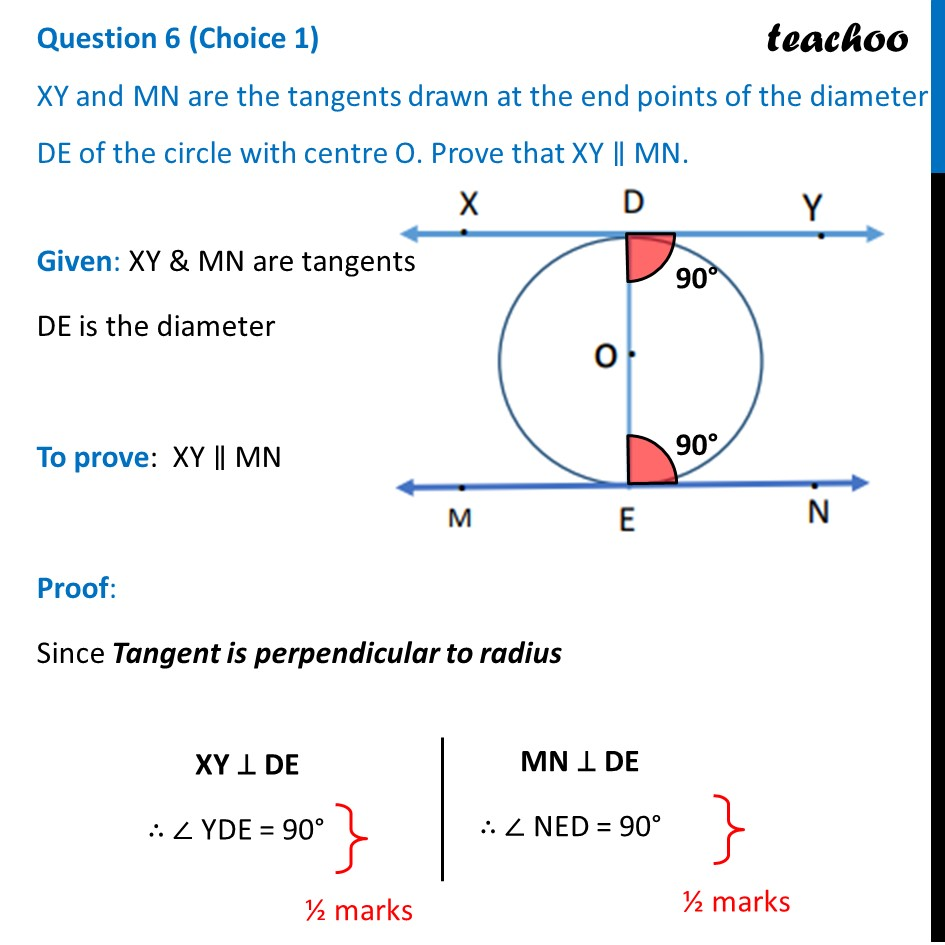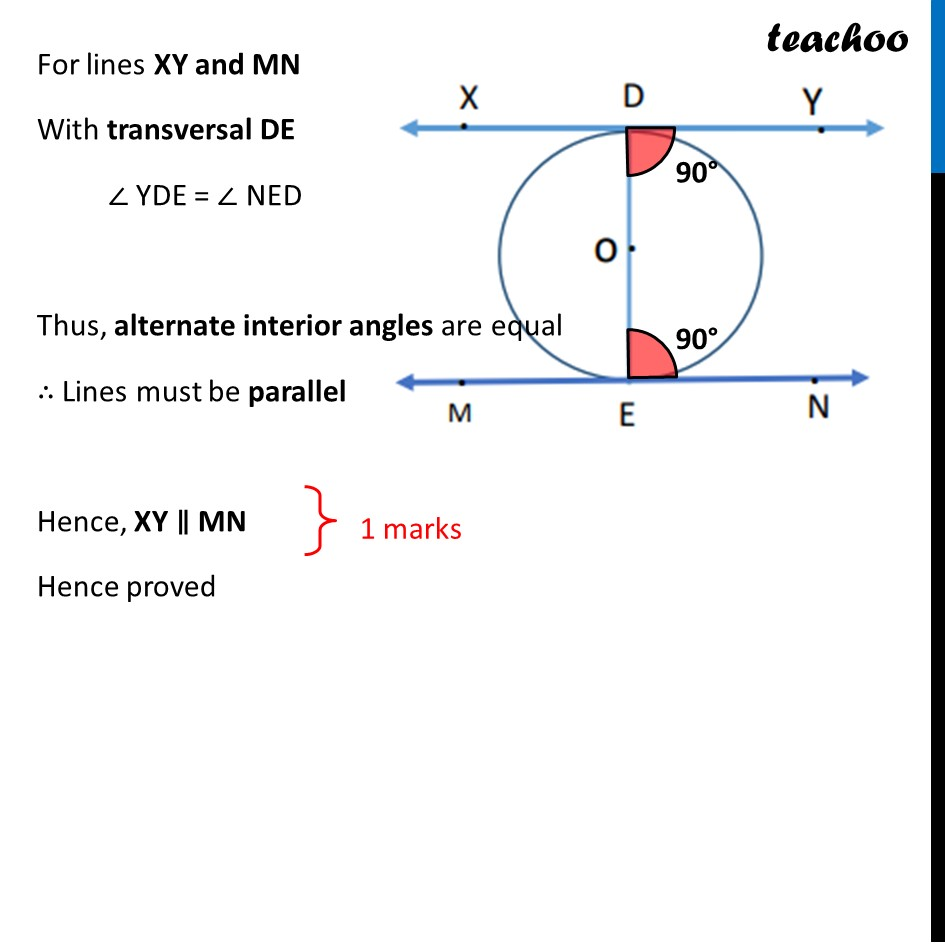CBSE Class 10 Sample Paper for 2022 Boards - Maths Basic [Term 2]

Class 10
Solutions of Sample Papers for Class 10 Boards

## XY and MN are the tangents drawn at the end points of the diameter DE of the circle with centre O. Prove that XY ∥ MN.

This question is similar to Ex 10.2, 9 Chapter 10 Class 10 - CirclesGet live Maths 1-on-1 Classs - Class 6 to 12

### Transcript

XY and MN are the tangents drawn at the end points of the diameter DE of the circle with centre O. Prove that XY ∥ MN. Given: XY & MN are tangents DE is the diameter To prove: XY ∥ MN Proof: Since Tangent is perpendicular to radius For lines XY and MN With transversal DE ∠ YDE = ∠ NED Thus, alternate interior angles are equal ∴ Lines must be parallel Hence, XY ∥ MN Hence proved